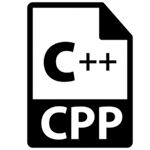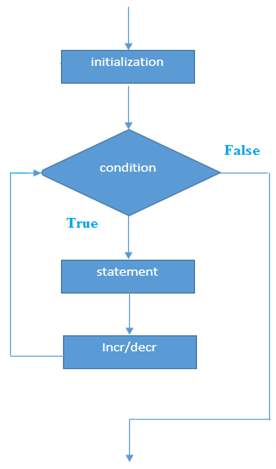# C++ for循环

C++ for循环用于重复程序的一部分几次(或多次)。 如果迭代次数是固定的，建议使用for循环，其次是while或do-while循环。

C++中的for循环与C/C＃中的for循环相同。 我们可以初始化变量，检查条件，然后增量/减量值。

for循环的语法如下所示

```for(initialization; condition; incr/decr){
//code to be executed
}```

for循环的流程图如下 -for循环的示例

```#include <iostream>
using namespace std;
int main() {
for(int i=1;i<=10;i++){
cout<<i <<"\n";
}
return 0;
}
```

```1
2
3
4
5
6
7
8
9
10
```

## C++嵌套for循环

C++嵌套for循环示例

```#include <iostream>
using namespace std;

int main () {
for(int i=1;i<=3;i++){
for(int j=1;j<=3;j++){
cout<<i<<" "<<j<<"\n";
}
}
return 0;
}
```

```1 1
1 2
1 3
2 1
2 2
2 3
3 1
3 2
3 3
```

## C++无限循环

```#include <iostream>
using namespace std;

int main () {
for (; ;)
{
cout<<"Infinitive For Loop"<<endl;
}
return 0;
}
```

```Infinitive For Loop
Infinitive For Loop
Infinitive For Loop
Infinitive For Loop
Infinitive For Loop
ctrl+c```